# 写在前面SIGGRAPH上的这个演讲讲的主要是3D动态云彩的渲染，适合于端游这样的大型游戏。后来在翻iq的博客的时候，发现偶像在2005年就写过一篇关于2D动态云模拟的文章，里面用到的算法相对来说简单许多，计算量也很少。这篇文章写于十年前，那时候电脑的计算资源非常有限，因此为了提升性能、减少内存占用等目的，提出了很多trick。尽管现在电脑的运算资源好了许多，但这些trick也没有因此退出舞台，而是可以作用到移动平台。这篇文章就是想要介绍一下iq那篇文章里提到的方法。

# 2D动态云彩

src="https://player.vimeo.com/video/153370722?color=c9ff23&title=0&byline=0&portrait=0" width="500" height="281" allowfullscreen="">

# 算法实现

## 创建噪声纹理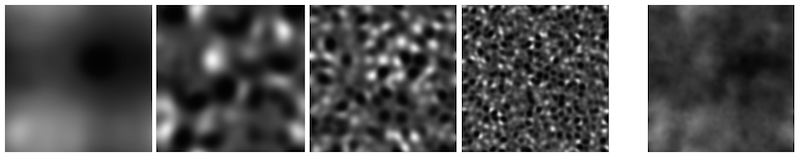sampler2D _Octave0;
sampler2D _Octave1;
sampler2D _Octave2;
sampler2D _Octave3;
float4 _Octave0_ST;
float4 _Octave1_ST;
float4 _Octave2_ST;
float4 _Octave3_ST;

v2f vert (appdata v) {
v2f o;
o.pos = mul(UNITY_MATRIX_MVP, v.vertex);
o.uv0.xy = TRANSFORM_TEX(v.texcoord, _Octave0) + _Time.x * 1.0 * _Speed * half2(1.0, 0.0);
o.uv0.zw = TRANSFORM_TEX(v.texcoord, _Octave1) + _Time.x * 1.5 * _Speed * half2(0.0, 1.0);
o.uv1.xy = TRANSFORM_TEX(v.texcoord, _Octave2) + _Time.x * 2.0 * _Speed * half2(0.0, -1.0);
o.uv1.zw = TRANSFORM_TEX(v.texcoord, _Octave3) + _Time.x * 2.5 * _Speed * half2(-1.0, 0.0);
return o;
}

fbm=0.5n0+0.52n1+0.53n2+0.54n3
<script type="math/tex; mode=display" id="MathJax-Element-1">fbm = 0.5 * n_0 + 0.5^2 * n_1 + 0.5^3 * n_2 + 0.5^4 * n_3</script>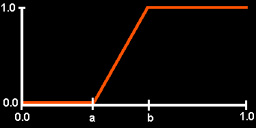float4 n0 = tex2D(_Octave0, i.uv0.xy);
float4 n1 = tex2D(_Octave1, i.uv0.zw);
float4 n2 = tex2D(_Octave2, i.uv1.xy);
float4 n3 = tex2D(_Octave3, i.uv1.zw);

float4 fbm = 0.5 * n0 + 0.25 * n1 + 0.125 * n2 + 0.0625 * n3;
fbm = (clamp(fbm, _Emptiness, _Sharpness) -  _Emptiness)/(_Sharpness - _Emptiness);

Pass {
// 渲染天空背景
...
}
Pass {
// 渲染云彩层
// 开启并设置混合系数，和上一个Pass的结果进行混合
Blend SrcAlpha OneMinusSrcAlpha

...

fixed4 frag (v2f i) : SV_Target {
fixed4 col;
...
col.rgb = _CloudColor.rgb;
col.a = fbm;
}
}

// 可以渲染多个云彩Pass
Pass {
// 同上一个Pass，但噪声纹理UV的移动速度和方向有所不同
}

iq指出，但如果直接这么混合的话会让整个效果看起来很平淡（flat），iq把raymarching的思想考虑进来，添加了太阳（或月亮）方向对云彩颜色的影响，让整个效果看起来更加立体。

## 添加raymarching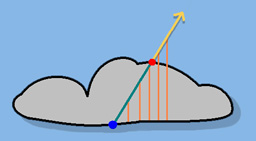fixed4 frag (v2f i) : SV_Target {
fixed4 col = 0;

float4 n0 = tex2D(_Octave0, i.uv0.xy);
float4 n1 = tex2D(_Octave1, i.uv0.zw);
float4 n2 = tex2D(_Octave2, i.uv1.xy);
float4 n3 = tex2D(_Octave3, i.uv1.zw);

float4 fbm = 0.5 * n0 + 0.25 * n1 + 0.125 * n2 + 0.0625 * n3;
fbm = (clamp(fbm, _Emptiness, _Sharpness) -  _Emptiness)/(_Sharpness - _Emptiness);

fixed4 ray = fixed4(0.0, 0.2, 0.4, 0.6);
fixed amount = dot(max(fbm - ray, 0), fixed4(0.25, 0.25, 0.25, 0.25));

col.rgb = amount * _CloudColor.rgb +  2.0 * (1.0 - amount) * 0.4;
col.a = amount * 1.5;

return col;
}

# 代码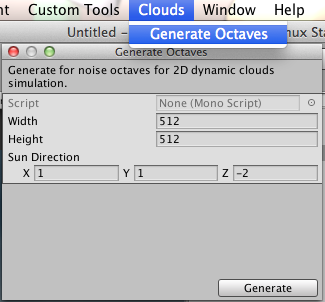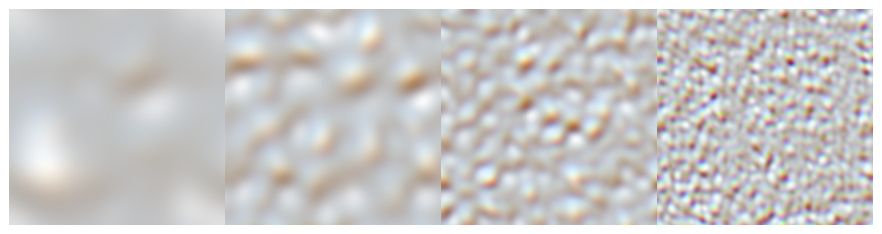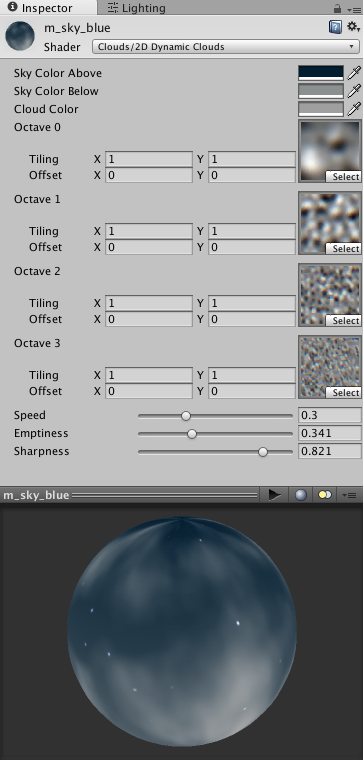# 写在最后09-26270
12-263997
01-073790